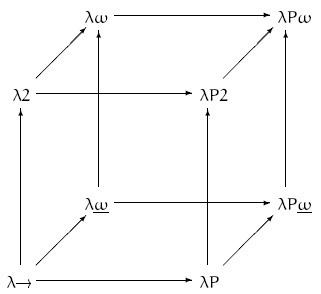# Lambda cube

Updated onIn mathematical logic and type theory, the λ-cube is a framework for exploring the axes of refinement in Coquand's calculus of constructions, starting from the simply typed lambda calculus (written as λ→ in the cube diagram to the right) as the vertex of a cube placed at the origin, and the calculus of constructions (higher-order dependently typed polymorphic lambda calculus; written as λPω in the diagram) as its diametrically opposite vertex. Each axis of the cube represents a new form of abstraction:

• Values depending on types, or polymorphism. System F, aka second order lambda calculus (written as λ2 in the diagram), is obtained by imposing only this property.
• Types depending on types, or type operators. Simply typed lambda calculus with type operators (λω in the diagram) is obtained by imposing only this property. Combined with System F it yields System Fω (written as λω without the underline in the diagram).
• Types depending on values, or dependent types. Imposing only this property yields λΠ (written as λP in the diagram), a type system closely related to LF.
• All eight calculi include the most basic form of abstraction, values depending on values, ordinary functions as in the simply typed lambda calculus. The richest calculus in the cube, with all three abstractions, is the calculus of constructions. All eight calculi are strongly normalizing.

Subtyping however is not represented in the cube, even though systems like F <: ω , known as higher-order bounded quantification, which combines subtyping and polymorphism are of practical interest, and can be further generalized to bounded type operators. Further extensions to F <: ω allow the definition of purely functional objects; these systems were generally developed after the lambda cube paper was published.

The idea of the cube is due to the mathematician Henk Barendregt (1991). The framework of pure type systems generalizes the lambda cube in the sense that all corners of the cube, as well as many other systems can be represented as instances of this general framework. This framework predates lambda cube by a couple of years. In his 1991 paper, Barendregt also defines the corners of the cube in this framework.

In his Habilitation à diriger les recherches Lambda-Prolog de A à Z... ou presque , Olivier Ridoux gives a cut-out template of the Lambda cube (p. 70) and also a dual representation of the cube as an octahedron, where the 8 vertices are replaced by faces, as well as a dual representation as a dodecahedron, where the 12 edges are replaced by faces.

## References

Similar Topics
Open Secret
Rob Ambrose
Margherita Rinaldi
Topics